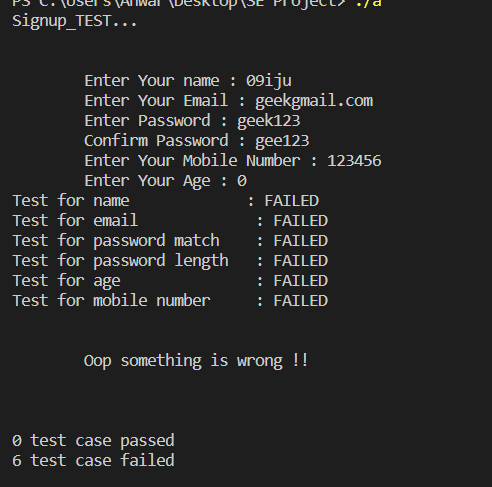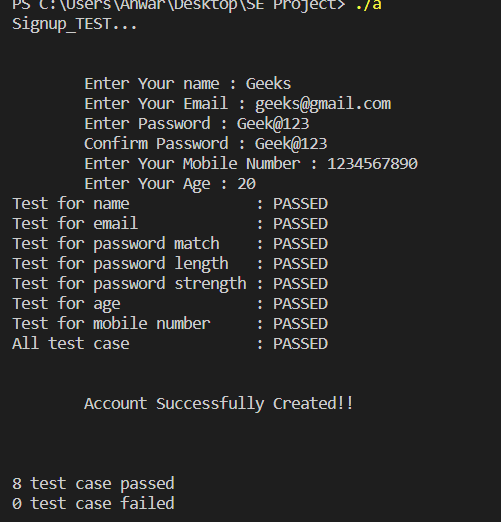Open in App
Not now

# Test Cases For Signup Page Using C Language

• Last Updated : 17 Jan, 2023

Prerequisite: Structure in C

In this article, we are going to check on how to check test cases for the signup page using C language. The signup page is where we enter data to create our account on any of the websites or applications.

A test case is a document that contains various sets of data, conditions to be performed, and the expected result for the particular condition, developed by the software testers to perform the execution of the particular part of the software to verify the working of the software against requirements. In order to verify a program, start by adding valid data to the input. Test data can include information like names, emails, passwords, mobile numbers, and ages. For multiple test cases, identifying data may become the longest step of the verification process. Identifying data can help understand what the program documents as a pass or a failure.

The data we need to enter on the signup page is:

1. User Name
2. Email
4. Contact number
5. Age

There are certain conditions for the data we are entering for example

1. The username must contain the alphabet.
2. Age must be greater than and not equal to 0.
3. Email must contain @, a comma, and the length should be greater than 5.
4. Passwords must contain a length between 8 to 12 with at least one uppercase, lowercase, number, and special character.
6. Mobile number should contain numbers and exactly 10 digits.

In this article, we will learn how to check test cases for the signup page.

“Test.h” Code:

## C

 `// C Program to write first program``// "test.h" file name``#include `` ` `typedef` `struct` `test_node test_node;`` ` `// Struct declared``struct` `test_node {``    ``test_node* next;``    ``void` `(*func)(``void``);``};`` ` `extern` `void` `add_test(test_node* tn);`` ` `#define test_assert(b)                                     \``    ``do` `{                                                   \``        ``if` `(b) {                                           \``            ``printf``(``"PASSED\n"``);                            \``            ``tests_passed++;                                \``        ``}                                                  \``        ``else` `{                                             \``            ``printf``(``"FAILED\n"``);                            \``            ``tests_failed++;                                \``        ``}                                                  \``    ``} ``while` `(0)`` ` `#define UNIT_TEST(f)                                       \``    ``static` `void` `f(``void``);                                   \``    ``static` `void` `__attribute__((constructor))               \``        ``__construct_##f(``void``)                              \``    ``{                                                      \``        ``static` `test_node tn;                               \``        ``tn.next = NULL;                                    \``        ``tn.func = f;                                       \``        ``add_test(&tn);                                     \``    ``}                                                      \``    ``static` `void` `__real_##f(``void``);                          \``    ``static` `void` `f(``void``)                                    \``    ``{                                                      \``        ``printf``(``"%s... "``, __func__);                        \``        ``fflush``(stdout);                                    \``        ``__real_##f();                                      \``    ``}                                                      \``    ``static` `void` `__real_##f(``void``)`` ` `int` `tests_passed = 0, tests_failed = 0;``test_node* start = 0;`` ` `void` `add_test(test_node* tn)``{``    ``test_node** current = &start;``    ``while` `(*current)``        ``current = &((*current)->next);``    ``*current = tn;``}`` ` `// Driver code``int` `main()``{``    ``test_node* current = start;``    ``while` `(current) {``        ``current->func();``        ``current = current->next;``    ``}``    ``int` `total_tests = tests_passed + tests_failed;``    ``if` `(total_tests == 0)``        ``printf``(``"No tests found\n"``);``    ``else``        ``printf``(``            ``"%i test case passed\n%i test case failed\n\n"``,``            ``tests_passed, tests_failed);``    ``return` `tests_failed > 0;``}`

Main Program: This is the main program that checks the data if we can create a signup page, also we are using a program written in another file “test.h”.

Signup Code:

## C

 `// C Program to check``// Test cases for signup page``#include "test.h"``#include ``#include ``#include ``#include `` ` `char` `temp_name, temp_password1;``char` `temp_password2, temp_email;``char` `temp_mobile;``int` `temp_age;`` ` `struct` `det {``    ``char` `uname;``    ``int` `age;``    ``char` `password;``    ``char` `email;``    ``char` `mobile;``};``int` `count, success, j;``struct` `det s;``UNIT_TEST(Signup_TEST)``{``    ``printf``(``"\n\n"``);``    ``printf``(``"\n\tEnter Your name : "``);``    ``scanf``(``"%s"``, temp_name);``    ``printf``(``"\tEnter Your Email : "``);``    ``scanf``(``"%s"``, temp_email);``    ``printf``(``"\tEnter Password : "``);``    ``scanf``(``"%s"``, temp_password1);``    ``printf``(``"\tConfirm Password : "``);``    ``scanf``(``"%s"``, temp_password2);``    ``printf``(``"\tEnter Your Mobile Number : "``);``    ``scanf``(``"%s"``, temp_mobile);``    ``printf``(``"\tEnter Your Age : "``);``    ``scanf``(``"%d"``, &temp_age);``    ``int` `i = 0, flag = 1;``    ``int` `check = 0;``    ``do` `{``        ``if` `(!((temp_name[i] >= ``'a'` `&& temp_name[i] <= ``'z'``)``//checking whether name contains any numbers``              ``|| (temp_name[i] >= ``'A'``                  ``&& temp_name[i] <= ``'Z'``))) {``            ``flag = 0;``            ``break``;``        ``}``        ``i = i + 1;``    ``} ``while` `(temp_name[i] != ``'\0'``);``    ``if` `(flag == 1) {``        ``check++;``        ``printf``(``"Test for name           : "``);``        ``test_assert(``true``);``    ``}``    ``else` `{`` ` `        ``printf``(``"Test for name          : "``);``        ``test_assert(``false``);``    ``}`` ` `    ``do` `{``        ``if` `(temp_email[i] == ``'@'` `|| temp_email[i] == ``'.'``) {``//checking email contains @ and . or not``            ``count++;``        ``}``        ``i = i + 1;``    ``} ``while` `(temp_email[i] != ``'\0'``);``    ``if` `(count >= 2 && ``strlen``(temp_email) >= 5) {``        ``check++;``        ``printf``(``"Test for email           : "``);``        ``test_assert(``true``);``    ``}``    ``else` `{`` ` `        ``printf``(``"Test for email           : "``);``        ``test_assert(``false``);``    ``}`` ` `    ``if` `(!``strcmp``(temp_password1, temp_password2)) {``//checking password and confirmpassword is same``        ``check++;``        ``printf``(``"Test for password match    : "``);``        ``test_assert(``true``);``    ``}``    ``else` `{`` ` `        ``printf``(``"Test for password match    : "``);``        ``test_assert(``false``);``    ``}`` ` `    ``if` `(``strlen``(temp_password1) >= 8``        ``&& ``strlen``(temp_password1) <= 12) {``// password must be greater than or equal to 8 and less than or equal to 12``        ``check++;``        ``printf``(``"Test for password length   : "``);``        ``test_assert(``true``);``    ``}``    ``else` `{`` ` `        ``printf``(``"Test for password length   : "``);``        ``test_assert(``false``);``    ``}``    ``if` `(``strlen``(temp_password1) >= 8``        ``&& ``strlen``(temp_password1) <= 12) {``        ``int` `caps = 0;``        ``int` `Small = 0;``        ``int` `numbers = 0;``        ``int` `special = 0;``        ``i = 0;``        ``do` `{``            ``if` `(temp_password1[i] >= ``'A'``//password must be contain capital letter``                ``&& temp_password1[i] <= ``'Z'``) {``                ``caps = caps + 1;``            ``}``            ``else` `if` `(temp_password1[i] >= ``'a'``//password must be contain small letter``                     ``&& temp_password1[i] <= ``'z'``) {``                ``Small = Small + 1;``            ``}``            ``else` `if` `(temp_password1[i] >= ``'0'``//password must be contain numbers``                     ``&& temp_password1[i] <= ``'9'``) {``                ``numbers = numbers + 1;``            ``}``            ``else` `if` `(temp_password1[i] == ``'@'``//password must be contain special character``                     ``|| temp_password1[i] == ``'&'``                     ``|| temp_password1[i] == ``'#'``                     ``|| temp_password1[i] == ``'*'``) {``                ``special = special + 1;``            ``}``            ``i = i + 1;``        ``} ``while` `(temp_password1[i] != ``'\0'``);``        ``if` `(caps >= 1 && Small >= 1 && numbers >= 1``            ``&& special >= 1) {``            ``check++;``            ``printf``(``"Test for password strength : "``);``            ``test_assert(``true``);``        ``}``        ``else` `{`` ` `            ``printf``(``"Test for password strength : "``);``            ``test_assert(``false``);``        ``}``    ``}`` ` `    ``if` `(temp_age > 0) {``//age must be greater than 0``        ``check++;``        ``printf``(``"Test for age           : "``);``        ``test_assert(``true``);``    ``}``    ``else` `{`` ` `        ``printf``(``"Test for age           : "``);``        ``test_assert(``false``);``    ``}``    ``if` `(``strlen``(temp_mobile) == 10) {``//contact number should be exact 10 digits``        ``do` `{``            ``if` `(temp_mobile[i] >= ``'0'``                ``&& temp_mobile[i] <= ``'9'``) {``                ``success = 1;``            ``}``            ``else` `{``                ``break``;``            ``}``            ``i = i + 1;``        ``} ``while` `(i < 10);``        ``if` `(success = 1) {``            ``check++;``            ``printf``(``"Test for mobile number     : "``);``            ``test_assert(``true``);``        ``}``        ``else` `{`` ` `            ``printf``(``"Test for mobile number     : "``);``            ``test_assert(``false``);``        ``}``    ``}``    ``else` `{`` ` `        ``printf``(``"Test for mobile number     : "``);``        ``test_assert(``false``);``    ``}``    ``i = 0;`` ` `    ``do` `{``        ``if` `(!(``strcmp``(temp_email, s[i].email)``              ``&& ``strcmp``(temp_password1, s[i].password))) {``            ``printf``(``"\n\n\tAccount Already Existed. Please "``                   ``"Login !!\n\n"``);``            ``flag = 0;``            ``break``;``        ``}``        ``i = i + 1;``    ``} ``while` `(i < 100);``    ``if` `(check == 7) {``        ``strcpy``(s[j].uname, temp_name);``        ``s[j].age = temp_age;``        ``strcpy``(s[j].password, temp_password1);``        ``strcpy``(s[j].email, temp_email);``        ``strcpy``(s[j].mobile, temp_mobile);``        ``j++;``        ``printf``(``"All test case           : "``);``        ``test_assert(``true``);``        ``printf``(``"\n\n\tAccount Successfully Created!!\n\n"``);``    ``}``    ``else` `{``        ``printf``(``"\n\n\tOop something is wrong !!\n\n"``);``    ``}``    ``printf``(``"\n\n"``);``}`

Output:Wrong Data entered and outputCorrect Data entered and output

My Personal Notes arrow_drop_up